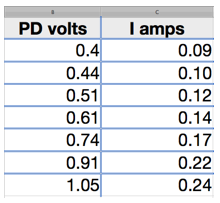Defining resistivity

Electrical resistivity is a property of a material. It does not depend upon the size or shape of the sample being tested. That could be a short thick bar or a long thin wire, it makes no difference as long as they are made from the same material. The resistivity is a measure of the amount that a material will resist the flow of an electric current.

The formal definition is that it the resistance of a one metre cube between opposite faces. However it is entirely impractical to test a shape like that so we use the formula that:

Resistivity =cross sectional area x resistance/length

Measuring resistivity

In order to calculate the resistivity we need to measure the length, diameter and resistance of our sample of wire.  The length is measured with a long tape and the diameter measured (in at least three places with accurate callipers or micrometer.

To measure the resistance of a sample wire it is best to measure a range of values of potential difference (volts) and current (amps).

The variable resistor is adjusted to give as wide a range as possible with values evenly spaced. From the range the graph plotted will provide a reliable value of resistance.The graph is plotted with PD, the controlling factor, on the x axis and current on the y axis. The line of best fit helps to even out small errors and would highlight any major mistakes in taking a result. The line does not have to pass through the origin.  The value of resistance V/I is the inverse of the gradient. Select any two convenient values, providing they employ most of the graph line, to make the calculation.

Resistivity calculation

Calculating resistivity

From the measurements taken for the sample of steel wire:

Length  2.78m

Diameter 0.38mm

Resistance 4.29 ohms